Question

# 1. The table below shows the expected rates of return for three stocks and their weight...

1. The table below shows the expected rates of return for three stocks and their weight in some portfolio:

 Stock A Stock B Stock C Expected return 0.05 0.03 0.13 Weight 0.5 0.2 0.3

a. What is the expected portfolio return?

2. You've assembled the following portfolio:

 Stock Expected return Portfolio weight 1 6.1% 30% 2 13.6% 3 17.3%

a. What is the weight for stock 3 if you want to achieve an expected portfolio return of 15%?

3. You've assembled the following portfolio:

 Stock Exp. return Investment 1 7.9% \$3,000 2 11.3% \$12,000 3 18.9% \$8,000

a. What is the expected return of the portfolio?

4. You've recorded the following historical annual returns for a stock:

 Year Return 1 8% 2 2% 3 -14% 4 3% 5 7%

a. What was the expected standard deviation of returns?

5. Which of the following statements are true about the capital allocation line (CAL)?

Check all that apply:

The CAL represents all portfolios with the same standard deviation but different expected returns.

An investor can increase their reward-to-volatility ratio by borrowing funds and investing more in risky assets (using leverage).

The slope of the capital allocation line is called the Sharpe ratio.

An investor can increase their expected return by borrowing funds and investing more in risky assets (using leverage).

The CAL represents all portfolios with the same expected return but different standard deviations.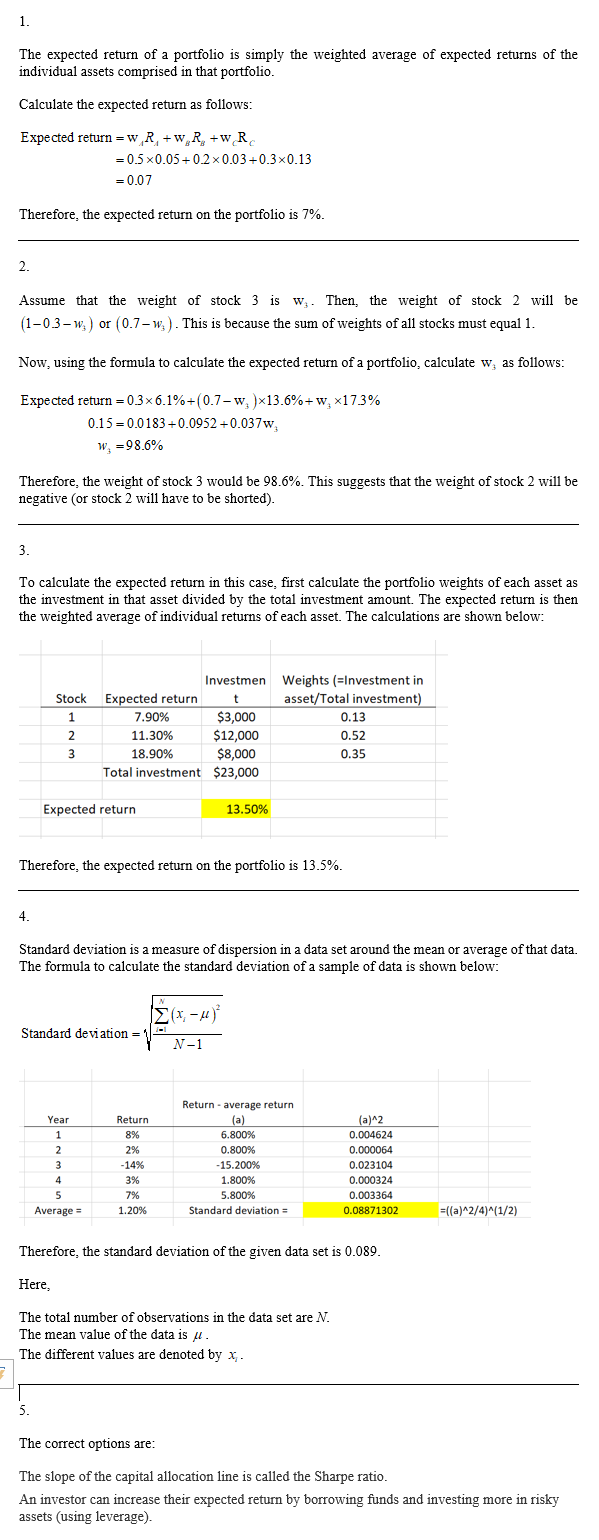#### Earn Coins

Coins can be redeemed for fabulous gifts.

Similar Homework Help Questions
• ### You manage a risky portfolio with an expected return of 12% and a standard deviation of 24%. Assume that you can invest...

You manage a risky portfolio with an expected return of 12% and a standard deviation of 24%. Assume that you can invest and borrow at a risk-free rate of 3%, using T-bills. a. Draw the Capital Allocation Line (CAL) for this combination of risky portfolio and risk-free asset. What is the Sharpe ratio of the risky portfolio? b. Your client chooses to invest 50% of their funds into your risky portfolio and 50% risk-free. What is the expected return and...

• ### 3. You have a risky portfolio that yields an expected rate of return of 15% with...

3. You have a risky portfolio that yields an expected rate of return of 15% with a standard deviation of 25%. Draw the CAL for an expected return/standard deviation diagram if the risk free rate is 5%. a. What is the slope of the CAL? b. If your coefficient of risk aversion is 5, how much should you invest in the risky portfolio? 4. A pension fund manager is considering three mutual funds. The first is a stock fund, the...

• ### answer all. For the next question, assume an investor with the following utility function U-E)-3/2) 12. To maximize her expected uility, she would choose the set with an espect rate of return of...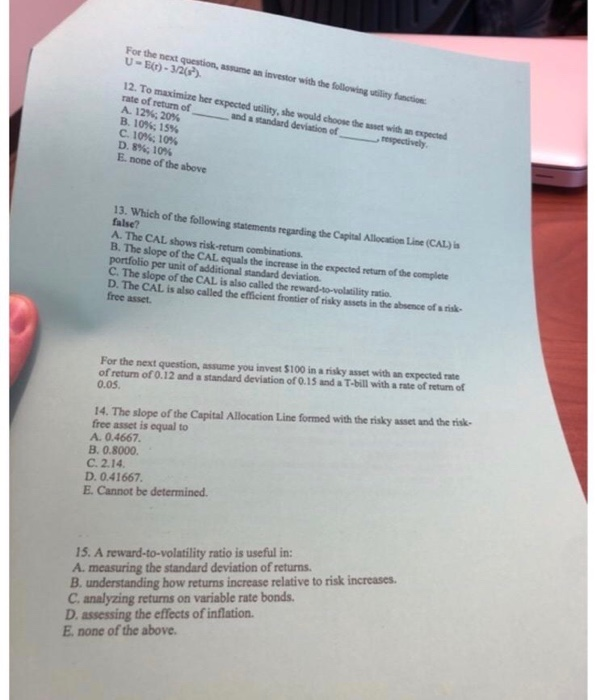answer all. For the next question, assume an investor with the following utility function U-E)-3/2) 12. To maximize her expected uility, she would choose the set with an espect rate of return of and a standard deviation ofrspectively A. 1296; 20% B. 10%; 15% C. 1056; 1056 D, 8%, 10% Е.none ofthe above 13. Which of the following statements regarding the Capital Allocation Line (CAL) false? A. The CAL shows risk-return combinations. B. The slope of the CAL equals the...

• ### Portfolio analvsis (8 points L3 points) Asset A has an expected return of 10% and a...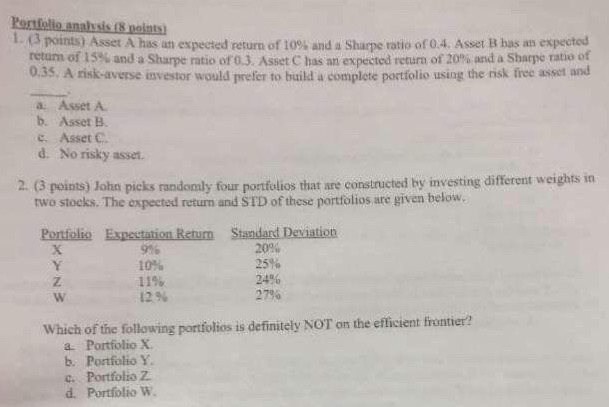Portfolio analvsis (8 points L3 points) Asset A has an expected return of 10% and a Sharpe ratio of 0.4. Asset B bas ans expectod retum of 15% and a Sharpe ratio of o3. Asset C has an expected return of 20% and a Sharpe ratio of 0.35. A risk-averse investor would prefer to build a complete portfolio using the risk free asset and a Asset A b. Asset B c Asset C d. No risky asset 2 (3 points)...

• ### 6. Consider the following information for Stocks 1 and 2: Expected Standard Stock Return Deviation 1...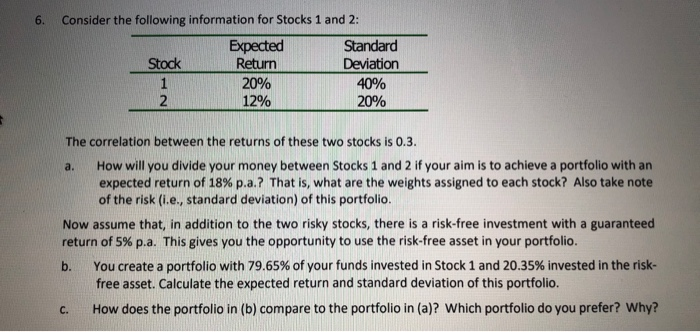6. Consider the following information for Stocks 1 and 2: Expected Standard Stock Return Deviation 1 20% 40% 2 12% 20% NE a. The correlation between the returns of these two stocks is 0.3. How will you divide your money between Stocks 1 and 2 if your aim is to achieve a portfolio with an expected return of 18% p.a.? That is, what are the weights assigned to each stock? Also take note of the risk (i.e., standard deviation) of...

• ### Intro You've assembled the following portfolio: Stock Expected return Portfolio weight 1 9.8% 30% 2 12% 3 16...

Intro You've assembled the following portfolio: Stock Expected return Portfolio weight 1 9.8% 30% 2 12% 3 16.8% Part 1 What is the weight for stock 3 if you want to achieve an expected portfolio return of 14%?

• ### 3. Which of the following statements are true? Please Explain. a. A lower allocation to the...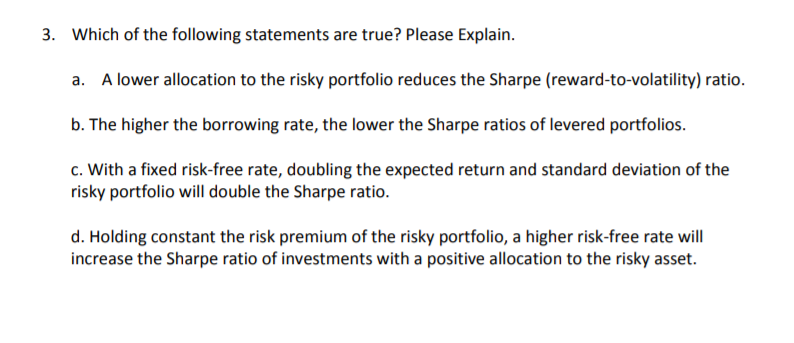3. Which of the following statements are true? Please Explain. a. A lower allocation to the risky portfolio reduces the Sharpe (reward-to-volatility) ratio. b. The higher the borrowing rate, the lower the Sharpe ratios of levered portfolios. c. With a fixed risk-free rate, doubling the expected return and standard deviation of the risky portfolio will double the Sharpe ratio. d. Holding constant the risk premium of the risky portfolio, a higher risk-free rate will increase the Sharpe ratio of investments...

• ### 1.3 (5 points) Two stocks have the following expected returns and standard deviations Stock Stock Expected...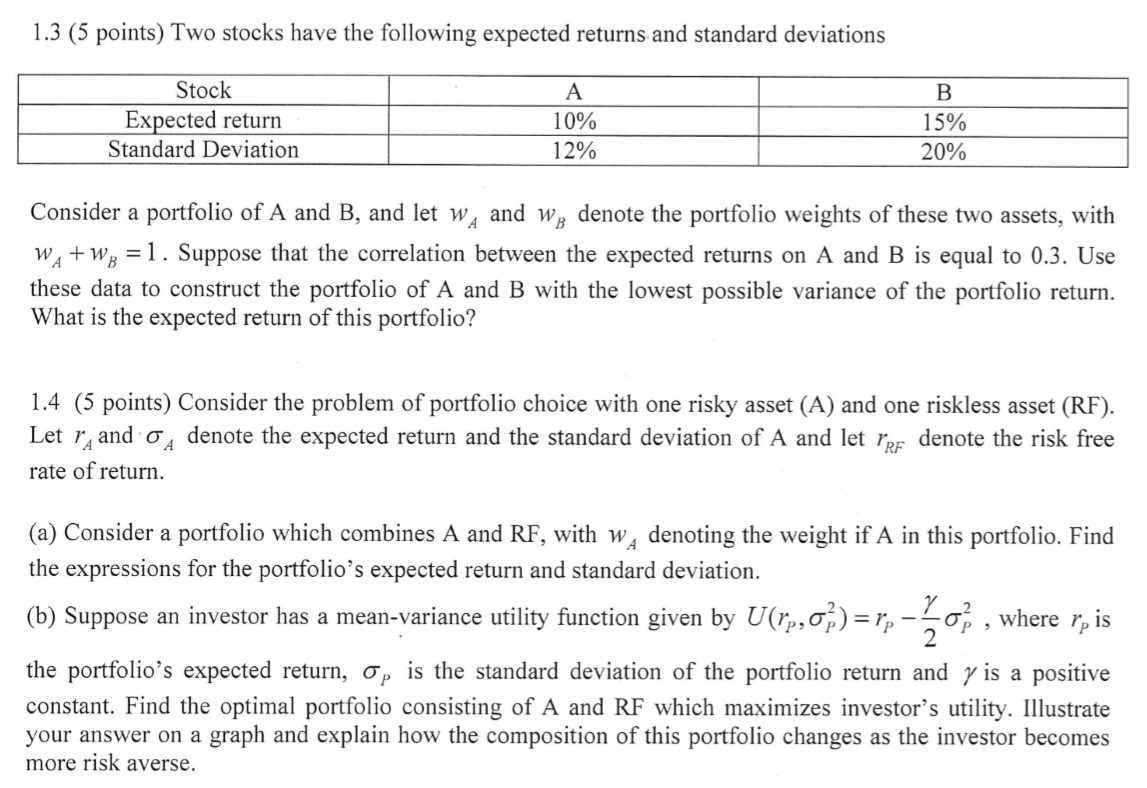1.3 (5 points) Two stocks have the following expected returns and standard deviations Stock Stock Expected return Standard Deviation A 10% 12% B 15% 20% Consider a portfolio of A and B, and let w, and wg denote the portfolio weights of these two assets, with W + W, =1. Suppose that the correlation between the expected returns on A and B is equal to 0.3. Use these data to construct the portfolio of A and B with the lowest...

• ### An investor has a risk aversion coefficient of 5. The expected return and standard deviation of...

An investor has a risk aversion coefficient of 5. The expected return and standard deviation of the optimal risky portfolio are 15% and 25%, respectively. If the Sharpe ratio of the optimal capital allocation line is 0.48, what is the proportion of the investor’s combined portfolio that should be invested in the risky portfolio that would maximise their utility?

• ### Problem 12 Intro You've assembled the following portfolio: Stock Expected return Portfolio weight 6.5% 30% 11.9% 18.3%...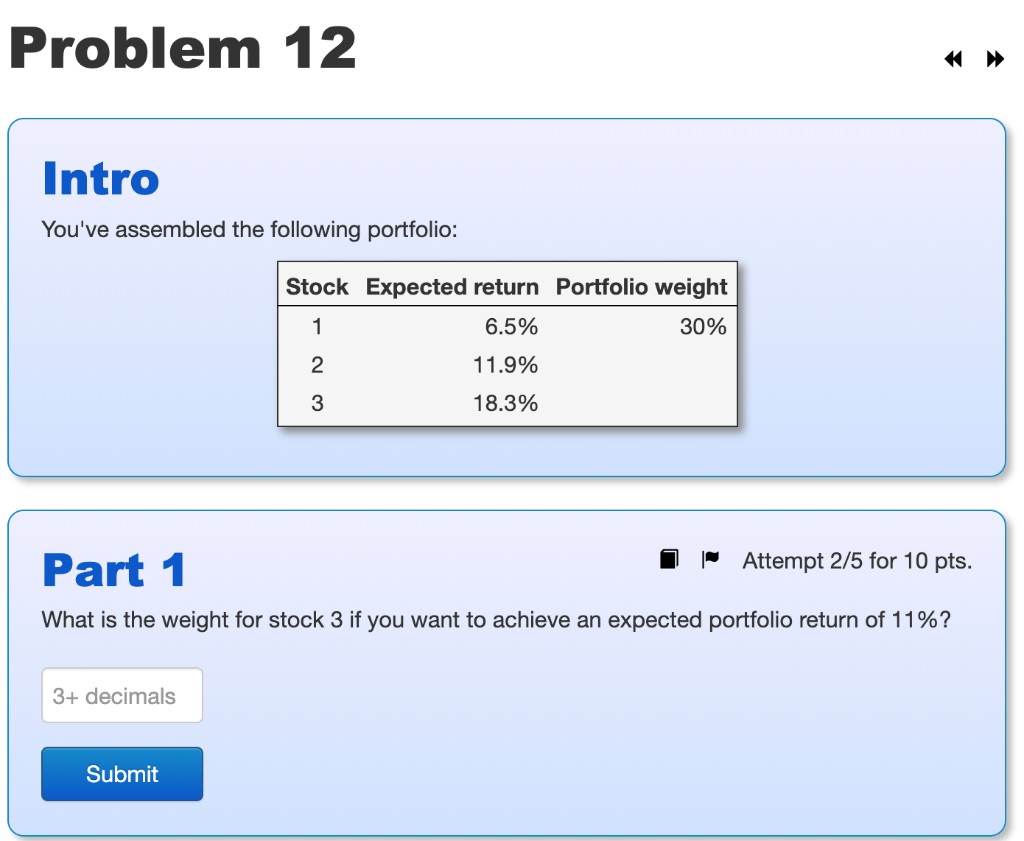Problem 12 Intro You've assembled the following portfolio: Stock Expected return Portfolio weight 6.5% 30% 11.9% 18.3% Part 1 1 Attempt 2/5 for 10 pts. What is the weight for stock 3 if you want to achieve an expected portfolio return of 11%? 3+ decimals Submit